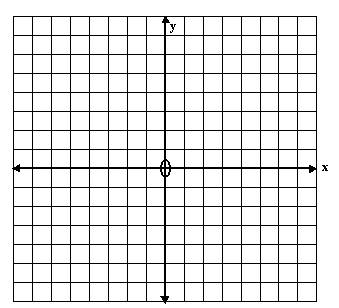# Plotting Co-ordinates, Graphing Tutorial

math tutorials > plotting co-ordinates

How to plot a graph using co-ordintates.

## What are Cartesian Co-ordinates?

Cartesian co-ordinates are given in the form (x,y), where the x-co-ordinate gives the point's position on the x-axis (horizontal axis) and the y-co-ordinate gives the point's position on the y-axis (vertical axis).

## Graphing problems for you to try

1. (1,0)
2. (7,6)
3. (-1,4)
4. (0,-2)
5. (-5,-1)
6. (4,-3)